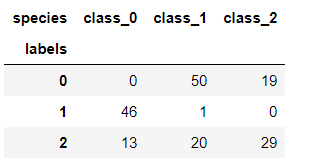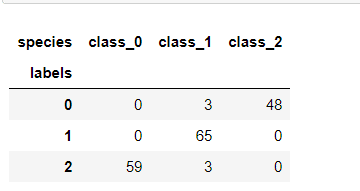# Transforming Features For Better Clustering | Python Unsupervised Learning -3

Hi, we continue where we left off on Unsupervised Learning. I recommend that you read our previous article before moving on to this article.

Python Unsupervised Learning -2

# Transforming Features For Better Clustering

Let’s look now at another dataset, the Piedmont wines dataset.

• 178 samples from 3 distinct varieties of red wine: Barolo, Grignolino and Barbera
• Features measure chemical composition e.g. alcohol content
• Visual properties like color intensity

#### Clustering the wines:

If you remember from our previous article, our cluster operations gave good results as a result of crosstabulation. Let’s write a new example with the Wine data and examine the results.

```from sklearn.cluster import KMeans
model = KMeans(n_clusters=3)
labels = model.fit_predict(wine.data)```

`df = pd.DataFrame({'labels':labels})`

```def species(theta):
if theta ==0:
return data.target_names
elif theta == 1:
return data.target_names
else:
return data.target_names```
`df["species"] = [species(theta) for theta in data.target]`

```cross_tab = pd.crosstab(df["labels"],df["species"])
cross_tab```As you can see, this time things haven’t worked out so well.  The KMeans clusters don’t correspond well with the wine varieties.

### Feature variances

• The wine features have very different variances!
• Variance of a feature measures spread of its values

# Transforming Features For Better Clustering

## StandartScaler

• In KMeans: feature variance = feature influence

To give every feature a chance the data needs to be transformed so that features have equal variance. This can be achieved with the StandartScaler from scikit-learn. It transforms every feature to have mean 0 and variance 1.

The resulting “standadized” features can be very informative.

Let’s practice,

```from sklearn.preprocessing import StandardScaler
scaler = StandardScaler()
scaler.fit(wine.data)
StandardScaler(copy=True, with_mean=True, with_std=True)
wine_scaled = scaler.transform(wine.data)```

The transform method can now be used to standardize any samples, either the same ones, or completely new ones.

## Similar Methods

• StandardScaler and KMeans have similar methods
• Use fit() / transform() with StandardScaler
• Use fit() / predict() with KMeans

# Piplines combine multiple steps

```from sklearn.preprocessing import StandardScaler
from sklearn.cluster import KMeans

scaler = StandardScaler()
kmeans = KMeans(n_clusters=3)

from sklearn.pipeline import make_pipeline
pipline = make_pipeline(scaler,kmeans)
pipline.fit(wine.data)````labels = pipline.predict(wine.data)`
```cross_tab = pd.crosstab(df["labels"],df["species"])
cross_tab```Checking the correspondence between the cluster labels and the wine varietes reveals that this new clustering, incorporating standardization, is fantastic.

Its three clusters correspond almost exactly to the three wine varieties.  This is a huge improvement on the clustering without standardization.

See you in the next article.

Python Unsupervised Learning -1

5,544 views last month,  2 views today